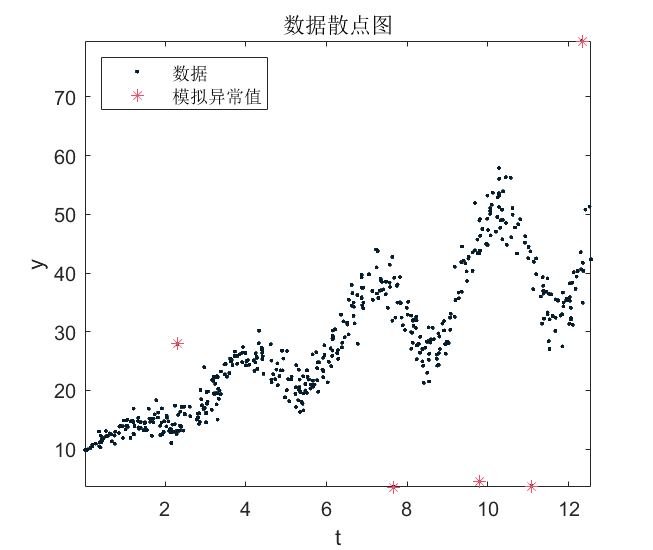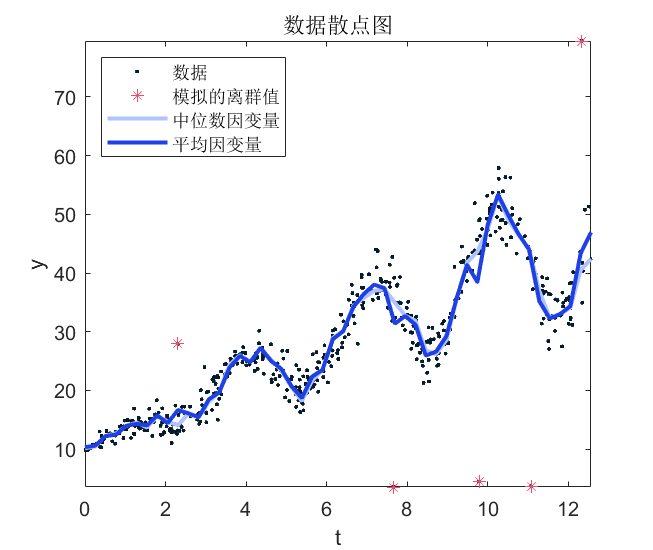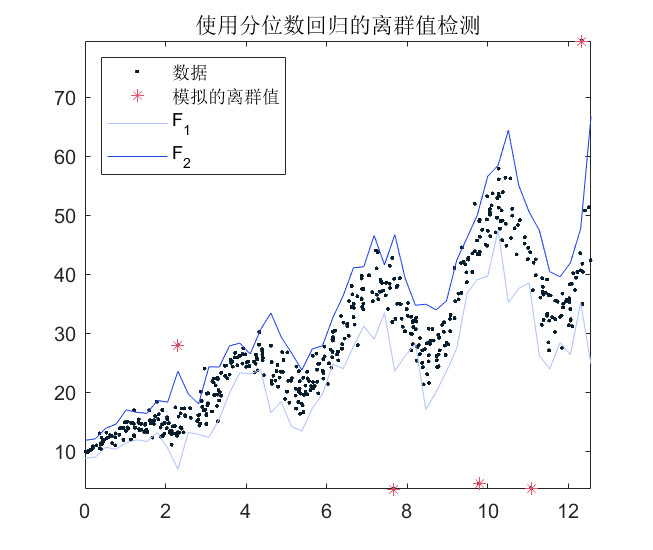# 生成数据

``````rng('default'); % 为保证重复性
randsample(linspace(0,4*pi,1e6),n,true)';
epsilon = randn(n,1).*sqrt((t+0.01)); ``````

`````` numOut = 5;
Tbl.y(idx) + randsample([-1 1],numOut,true)'.*(0.9*Tbl.y(idx)); ``````

``````​
plot(Tbl.t,Tbl.y,'.');
plot(Tbl.t(idx),Tbl.y(idx),'*');
title('数据散点图');
legend('数据','模拟异常值','Location','NorthWest');

​``````# 生成分位数随机森林

``Tree(200,'y','regression');``

`返回是一个TreeBagger集合。`

# 预测条件四分位数和四分位数区间

``````linspace(0,4*pi,50)';
quantile(pred,'Quantile');``````

# R语言用LOESS(局部加权回归)季节趋势分解（STL）进行时间序列异常检测

quartile是一个500 × 3的条件四分位数矩阵。行对应于t中的观测值，列对应于概率。

``````plot(pred,[quartiles(:,2) meanY]);
legend('数据','模拟的离群值','中位数因变量','平均因变量',... ``````

### 随时关注您喜欢的主题``````​

iqr = quartiles(:,3) - quartiles(:,1);
f1 = quartiles(:,1) - k*iqr;

​``````

# 将观测结果与边界进行比较

`````` plot(Tbl.t,Tbl.y,'.');
legend('数据','模拟的离群值','F_1','F_2');
title('使用分位数回归的离群值检测') ``````

# 所有模拟的异常值都在[F1，F2]之外，一些观测值也在这个区间之外。Kaizong Ye拓端研究室（TRL）的研究员。

​非常感谢您阅读本文，如需帮助请联系我们！QQ在线咨询

15121130882

0571-63341498

## 关注有关新文章的微信公众号

This will close in 0 seconds